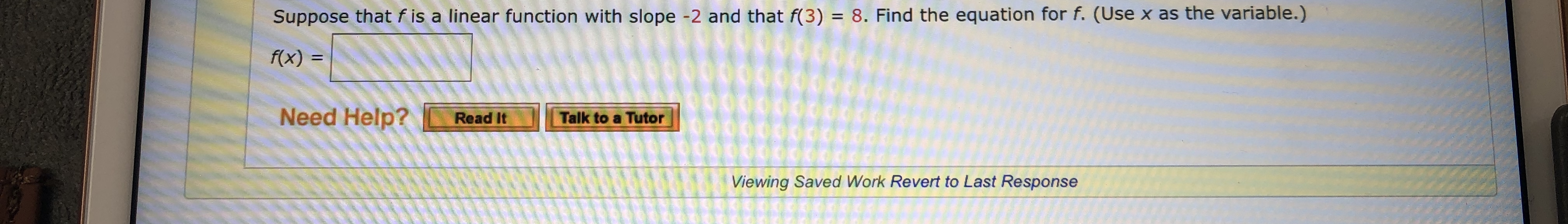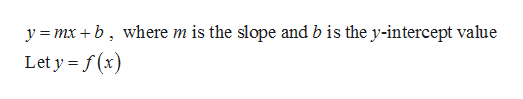# Suppose that f is a linear function with slope -2 and that f(3) = 8. Find the equation for f. (Use x as the variable.)f(x)pooGNeed Help?Talk to a TutorRead ItViewing Saved Work Revert to Last Response

Question

What are the blanks?help_outlineImage TranscriptioncloseSuppose that f is a linear function with slope -2 and that f(3) = 8. Find the equation for f. (Use x as the variable.) f(x) pooG Need Help? Talk to a Tutor Read It Viewing Saved Work Revert to Last Response fullscreen
check_circleExpert Solution
Step 1

The slope-intercept form of a linear equation is given by,help_outlineImage Transcriptionclosey mxb where m is the slope and b is the y-intercept value Let y f(x) fullscreen
Step 2

Given that, f is a linear function with slope (-2), which is written as,

Step 3

The function value of f, when x takes the value 3 is given as 8. Usi...

### Want to see the full answer?

See Solution

#### Want to see this answer and more?

Solutions are written by subject experts who are available 24/7. Questions are typically answered within 1 hour*

See Solution
*Response times may vary by subject and question
Tagged in

### Equations and In-equations